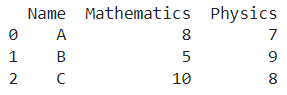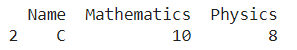# How to Filter Pandas DataFrame By Substring Criteria?

A DataFrame is the primary data structure of the Pandas library in Python and is commonly used for storing and working with tabular data.

A common operation that could be performed on such data is to filter the DataFrame by substring criteria to extract useful information out of it.

To start working with Pandas, we first need to import it :

``import pandas as pd``

## Running Example

Let us understand this operation with the help of an example. Consider the following DataFrame containing 3 students with names A, B, and C and their corresponding marks (out of 10) for two subjects, Mathematics and Physics.Code snippet for generating the above DataFrame :

``````import pandas as pd

# Dictionary for our data
data = {'Name' : ['A', 'B', 'C'], 'Mathematics' : [8, 5, 10], 'Physics' : [7, 9, 8]}

# DataFrame for the dictionary
df = pd.DataFrame(data)

# Printing the DataFrame
print(df)``````

Here, data is a dictionary we created to initialize the DataFrame.

For this, we use the DataFrame() function of the Pandas library which takes the dictionary as an argument and returns the required DataFrame.

Now, let’s say we need to filter the DataFrame by substring criteria, say the entry in the name column should have C as a substring.

The resulting output would look like this :Let us look at different ways of performing this operation on a given DataFrame :

## 1. Using the str.contains() function to filter data in pandas dataframe

In this method, we use the str.contains() function to filter a pandas dataframe, i.e. get desired rows based on substring criteria.

We use df[‘Name’] to access the name column, to which we apply the .str.contains() function.

We pass C as a parameter to this function to specify the required substring for filtering.

This returns a filter that could then be used with the help of square brackets to filter out the desired rows of the dataframe.

Let us take a look at the corresponding code snippet and generated output for this method :

``````# Importing required libraries
import pandas as pd

# Dictionary for our data
data = {'Name' : ['A', 'B', 'C'], 'Mathematics' : [8, 5, 10], 'Physics' : [7, 9, 8]}

# DataFrame for the dictionary
df = pd.DataFrame(data=data)

# Performing the operation
df = df[df['Name'].str.contains('C')]

# Printing
print(df)``````

Output :Instead of using df[‘Name’] to access the name column, we could also simply use the dot operator in the form of df.Name to get the same result.

Let us take a look at the corresponding code snippet and generated output for this method :

``````# Importing required libraries
import pandas as pd

# Dictionary for our data
data = {'Name' : ['A', 'B', 'C'], 'Mathematics' : [8, 5, 10], 'Physics' : [7, 9, 8]}

# DataFrame for the dictionary
df = pd.DataFrame(data=data)

# Performing the operation
df = df[df.Name.str.contains('C')]

# Printing
print(df)``````

Output :By using the == True inside the filter in the manner as shown in the example below also gives us the same result.

Let us take a look at the corresponding code snippet and generated output for this method :

``````# Importing required libraries
import pandas as pd

# Dictionary for our data
data = {'Name' : ['A', 'B', 'C'], 'Mathematics' : [8, 5, 10], 'Physics' : [7, 9, 8]}

# DataFrame for the dictionary
df = pd.DataFrame(data=data)

# Performing the operation
df = df[df.Name.str.contains('C') == True]

# Printing
print(df)``````

Output :Setting the parameter regex as False inside the str.contains() function gives us faster results.

The running time improvement might not be apparent in the case of a dataframe with a relatively smaller size such as the one that we are considering.

In much larger dataframes, this faster running time could be very helpful.

Let us take a look at the corresponding code snippet and generated output for this method :

``````# Importing required libraries
import pandas as pd

# Dictionary for our data
data = {'Name' : ['A', 'B', 'C'], 'Mathematics' : [8, 5, 10], 'Physics' : [7, 9, 8]}

# DataFrame for the dictionary
df = pd.DataFrame(data=data)

# Performing the operation
df = df[df.Name.str.contains('C', regex = False)]

# Printing
print(df)``````

Output :## Conclusion

In this topic, we have learned to filter an existing Pandas DataFrame by substring criteria, following a running example of test scores of students in different subjects, thus giving us an intuition of how this concept could be applied in real-world situations. Feel free to reach out to info.javaexercise@gmail.com in case of any suggestions.

Trending